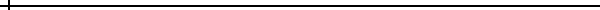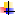### How to Use this Applet

The two green curves are parallel sinusoidal waveforms that have identical wavelengths, amplitudes, and phases when the applet initializes. The blue sinusoidal waveform at the bottom is the sum of the two green parallel waveforms. You can change the phase of the green sinusoidal waveforms by dragging the circles at the left end of the waveform. You can change the wavelength and the amplitude by dragging the other two circles. The sliders to the right of the waveforms offer alternative means of making similar changes.

To animate the waveforms, click on the animate checkbox, and to stop the animation, click again. In order to change the speed of the animation of the two green waveforms, you can use the sliders on the lower right, but you must halt the animation in order to adjust the speed. Once your speeds are selected, start the animation again. The speeds are actually phase velocity. In other words, when the two green waveforms animate at equal speeds, each one will advance by an equal number of wavelengths during a given amount of time. Therefore, if they are set to the same speed, but different wavelengths, the waveform with the longer wavelength will advance faster than the other one.

### Mathematical ConceptsSinusoidal waveformsWaveform amplitudeWavelengthWaveform summationPhase relationships